# 11.3 Discussion and Exercises

Sorting is the fundamental algorithmic problem in computer science, and it has a long history. Knuth  attributes the merge-sort algorithm to von Neumann (1945). Quicksort is due to Hoare . The original heap-sort algorithm is due to Williams , but the version presented here (in which the heap is constructed bottom-up intime) is due to Floyd . Lower-bounds for comparison-based sorting appear to be folklore. The following table summarizes the performance of these comparison-based algorithms:

 comparisons in-place Merge-sort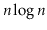worst-case No Quicksort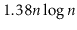expected Yes Heap-sort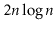worst-case Yes

Each of these comparison-based algorithms has its advantages and disadvantages. Merge-sort does the fewest comparisons and does not rely on randomization. Unfortunately, it uses an auxilliary array during its merge phase. Allocating this array can be expensive and is a potential point of failure if memory is limited. Quicksort is an in-place algorithm and is a close second in terms of the number of comparisons, but is randomized, so this running time is not always guaranteed. Heap-sort does the most comparisons, but it is in-place and deterministic.

There is one setting in which merge-sort is a clear-winner; this occurs when sorting a linked-list. In this case, the auxiliary array is not needed; two sorted linked lists are very easily merged into a single sorted linked-list by pointer manipulations (see Exercise 11.2).

The counting-sort and radix-sort algorithms described here are due to Seward [66, Section 2.4.6]. However, variants of radix-sort have been used since the 1920s to sort punch cards using punched card sorting machines. These machines can sort a stack of cards into two piles based on the existence (or not) of a hole in a specific location on the card. Repeating this process for different hole locations gives an implementation of radix-sort.

Finally, we note that counting sort and radix-sort can be used to sort other types of numbers besides non-negative integers. Straightforward modifications of counting sort can sort integers, in any interval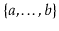, in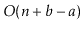time. Similarly, radix sort can sort integers in the same interval in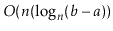time. Finally, both of these algorithms can also be used to sort floating point numbers in the IEEE 754 floating point format. This is because the IEEE format is designed to allow the comparison of two floating point numbers by comparing their values as if they were integers in a signed-magnitude binary representation .

Exercise 11..1   Illustrate the execution of merge-sort and heap-sort on an input array containing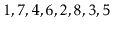. Give a sample illustration of one possible execution of quicksort on the same array.

Exercise 11..2   Implement a version of the merge-sort algorithm that sorts a DLList without using an auxiliary array. (See Exercise 3.13.)

Exercise 11..3   Some implementations of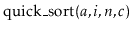always use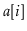as a pivot. Give an example of an input array of length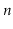in which such an implementation would perform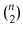comparisons.

Exercise 11..4   Some implementations ofalways use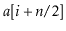as a pivot. Given an example of an input array of lengthin which such an implementation would performcomparisons.

Exercise 11..5   Show that, for any implementation ofthat chooses a pivot deterministically, without first looking at any values in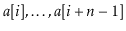, there exists an input array of lengththat causes this implementation to performcomparisons.

Exercise 11..6   Design a Comparator,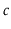, that you could pass as an argument toand that would cause quicksort to performcomparisons. (Hint: Your comparator does not actually need to look at the values being compared.)

Exercise 11..7   Analyze the expected number of comparisons done by Quicksort a little more carefully than the proof of Theorem 11.3. In particular, show that the expected number of comparisons is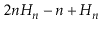.

Exercise 11..8   Describe an input array that causes heap sort to perform at least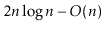comparisons. Justify your answer.

Exercise 11..9   Find another pair of permutations of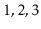that are not correctly sorted by the comparison tree in Figure 11.6.

Exercise 11..10   Prove that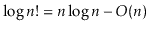.

Exercise 11..11   Prove that a binary tree with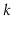leaves has height at least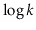.

Exercise 11..12   Prove that, if we pick a random leaf from a binary tree withleaves, then the expected height of this leaf is at least.

Exercise 11..13   The implementation of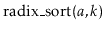given here works when the input array,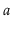contains only integers.

opendatastructures.org